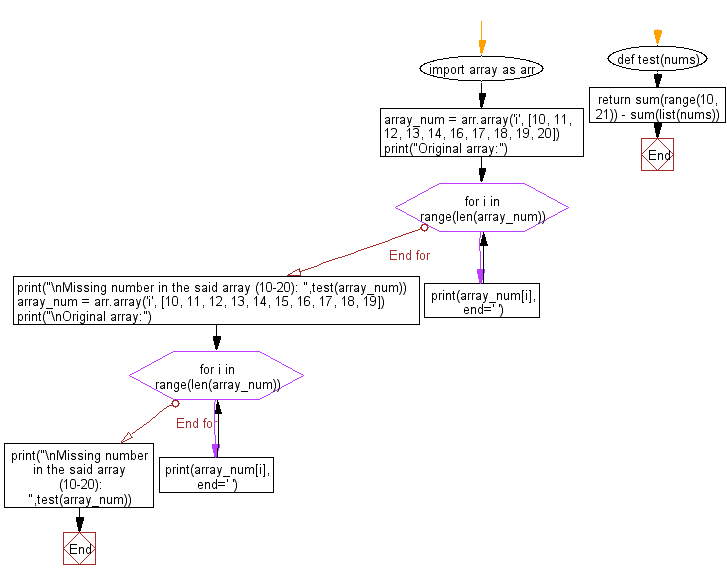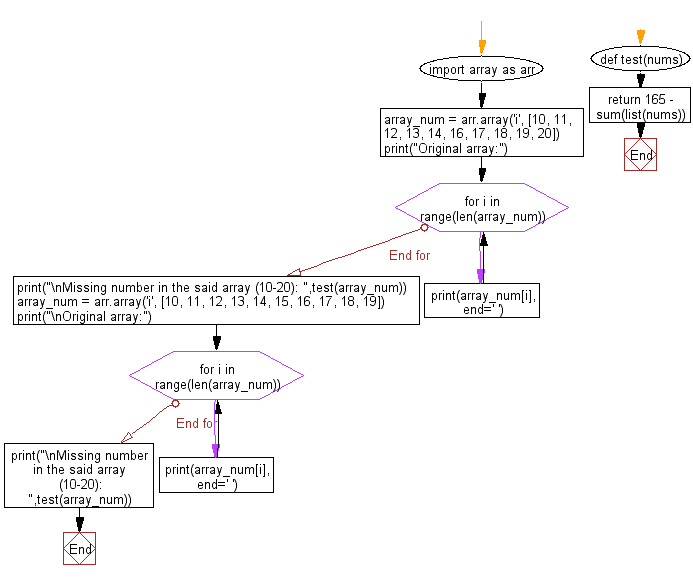﻿ Python: Find the missing number in a given array of numbers between 10 and 20 - w3resource

# Python: Find the missing number in a given array of numbers between 10 and 20

## Python: Array Exercise-24 with Solution

Write a Python program to find the missing number in a given array of numbers between 10 and 20.

Sample Solution-1:

Python Code:

``````import array as arr
def test(nums):
return sum(range(10, 21)) - sum(list(nums))

array_num = arr.array('i', [10, 11, 12, 13, 14, 16, 17, 18, 19, 20])
print("Original array:")
for i in range(len(array_num)):
print(array_num[i], end=' ')
print("\nMissing number in the said array (10-20): ",test(array_num))

array_num = arr.array('i', [10, 11, 12, 13, 14, 15, 16, 17, 18, 19])
print("\nOriginal array:")
for i in range(len(array_num)):
print(array_num[i], end=' ')
print("\nMissing number in the said array (10-20): ",test(array_num))
```
```

Sample Output:

```Original array:
10 11 12 13 14 16 17 18 19 20
Missing number in the said array (10-20):  15

Original array:
10 11 12 13 14 15 16 17 18 19
Missing number in the said array (10-20):  20
```

Flowchart:Sample Solution-2:

Python Code:

``````import array as arr
def test(nums):
return 165 - sum(list(nums))

array_num = arr.array('i', [10, 11, 12, 13, 14, 16, 17, 18, 19, 20])
print("Original array:")
for i in range(len(array_num)):
print(array_num[i], end=' ')
print("\nMissing number in the said array (10-20): ",test(array_num))

array_num = arr.array('i', [10, 11, 12, 13, 14, 15, 16, 17, 18, 19])
print("\nOriginal array:")
for i in range(len(array_num)):
print(array_num[i], end=' ')
print("\nMissing number in the said array (10-20): ",test(array_num))
``````

Sample Output:

```Original array:
10 11 12 13 14 16 17 18 19 20
Missing number in the said array (10-20):  15

Original array:
10 11 12 13 14 15 16 17 18 19
Missing number in the said array (10-20):  20
```

Flowchart:Python Code Editor: